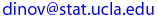Ivo Dinov
UCLA Statistics, Neurology, LONI
 Courses SOCR Ivo Dinov's Home SiteMap Software Contact

##### Interactive & Computational Probability

Text & Syllabus

 Instructor: Ivo D. Dinov, Ph.D. Assistant Professor of Statistics and Neurology UCLA School of Medicine E-mail: dinov@stat.ucla.edu

Textbook:
Instructor's Notes and SOCR resource pages

Overall Description:

Lecture, three hours; discussion, one hour. Requisites: Mathematics 31A, Program in Computing 10A.
Basic introductory probability topics in interactive problem-driven manner. Various applets, interfaces, and demonstrations used to illustrate fundamental properties of distributions, random number generation, combinatorics, expectation, variability, and sampling. Assignment of projects that require light computer programming. Emphasis on practical description, utilization, and graphical presentation of various probabilistic modeling techniques. P/NP or letter grading.

Tentative schedule of topics to be covered
1. Introduction - Probability, data analysis, sampling theory and statistical inference.
2. Basic Probability
• Probability models, sample space, events, “some” algebra of events
• Probability axioms, combinatorial problems, conditional probability, independent events, Bayes’ rule, Bernoulli trials.
• Discrete random variables, probability mass function, cumulative distribution functions, special discrete distributions (Bernoulli, Binomial, geometric, negative binomial, poisson, hypergeometric, discrete uniform), independent random variables.
• Continuous random variables, probability density function, cumulative distribution functions, special continuous distributions (exponential, normal, uniform, Pareto, maybe another one), distribution of sums of random variables, functions of normal random variables. A little bit of joint distribution of two random variables for the discrete and continuous case.
• Expectation, moments of a random variable, expected value of random variables with the distributions seen earlier
• Central Limit Theorem (CLT), Law of Large Numbers (LLN).
• Conditional distribution and expectations.
• Brief, very brief mention of stochastic processes, like queues, Markov models, etc…

3. Descriptive Data Analysis of random samples
• What is a random sample? Examples of samples arising from different probability models. Data not coming from random samples. Design of experiments.
• Types of data we encounter: measuring, counting, classifying.
• Sample Histograms and box plots for numerical variables
• Descriptive statistics of a sample: Sample mean, sample median, sample quartiles, interquartile range, range, sample proportion, variance and standard deviation
• Descriptive comparison of two groups
• Scatter plots
• Sample covariance and correlations
• Descriptive regression analysis

4. Sampling and back to probability
• Sample statistic
• Independent, dependent and random samples
• The expected value and variance of the sample mean
• The expected value and variance of the sample proportion
• Unbiasedness, precision, accuracy, consistency, efficiency of estimators
• Law of Averages
• Central Limit Theorem for the sample mean
• Central Limit theorem from sample proportion.

5. Statistical Inference
• Parameter estimation. Maximum likelihood estimation
• Confidence Intervals for the model mean
• Confidence Intervals for the model proportion
• Hypothesis testing for the model mean
• Hypothesis testing for the model proportion
• Confidence intervals and hypothesis testing for the difference of two mean and the same for the difference of two proportions.

 Ivo Dinov's Home http://www.stat.ucla.edu/~dinov Visitor number, since Jan. 01, 2002 © I.D.Dinov 1997-2006
Last modified on by